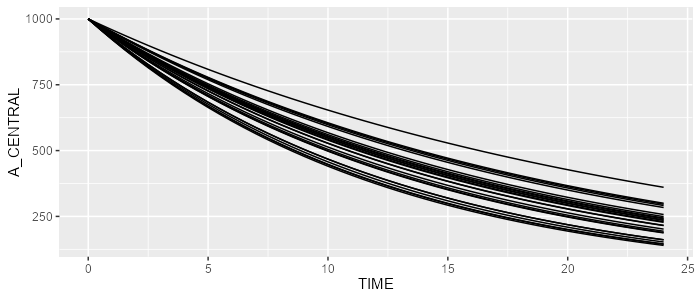# Create a minimalist model

### Prerequisite

For this exercise, we’ll need the campsismod package. This package can be loaded as follows:

library(campsismod)

### Create a minimalist model in the Notepad++ editor

Assume a very simple 1-compartment PK model with first-order eliminate rate K. Say this parameter has a typical value of log(2)/12≈0.06 (where 12 is the elimination half life) and has 15% CV. Let’s also initiate the central compartment to 1000.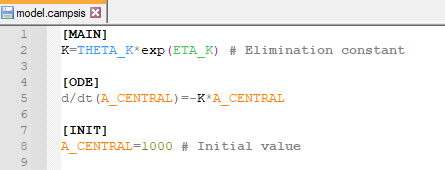Let’s now create our theta.csv with our single parameter K as follows: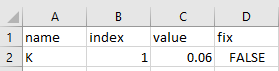Edition of THETA’s in Excel

And finally, let’s also create our omega.csv to include inter-individual variability on K: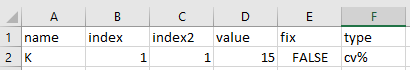Edition of OMEGA’s in Excel

This model can now be loaded by campsismod

model <- read.campsis("resources/minimalist_model/")
## Warning in read.allparameters(folder = folder): No file 'sigma.csv' could be
## found.

Let’s simulated this model in CAMPSIS:

library(campsis)
results <- model %>% simulate(dataset=dataset, seed=1)
spaghettiPlot(results, "A_CENTRAL")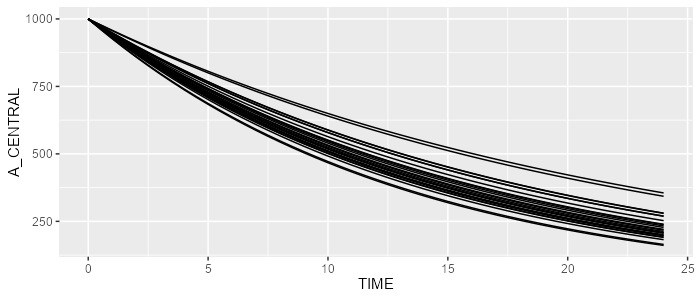### Create the same model programmatically

The same model can be created programmatically. First, let’s create an empty CAMPSIS model.

model <- CampsisModel()

Then, let’s define the equation of our model parameter K.

model <- model %>% add(Equation("K", "THETA_K*exp(ETA_K)"))

We can add an ordinary differential equation as follows:

model <- model %>% add(Ode("A_CENTRAL", "-K*A_CENTRAL"))

We can init the central compartment as well on the fly:

model <- model %>% add(InitialCondition(compartment=1, "1000"))

Finally, let’s define our THETA_K and ETA_K:

model <- model %>% add(Theta("K", value=0.06))
model <- model %>% add(Omega("K", value=15, type="cv%"))

This model can simulated by CAMPSIS as well. Powerful, isn’t it?

library(campsis)
spaghettiPlot(results, "A_CENTRAL")